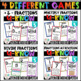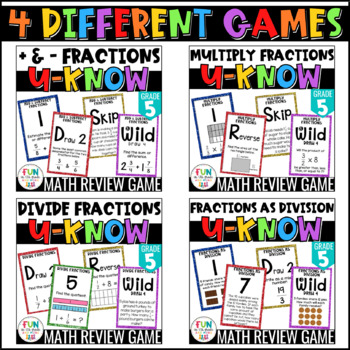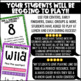# Fraction Games | U-Know Fraction Review Games MINI Bundle {5th Grade}Subject
Resource Type
File Type
Zip (67 MB|140 pages)
Standards
\$12.75
Bundle
List Price:
\$15.00
You Save:
\$2.25
Products in this Bundle (4)
1. Adding and subtracting fractions is important for you to teach and for students to learn! Let them practice with this fun and engaging game that will make the skill of practicing adding and subtracting fraction something students WANT to do! Works great for math centers / stations, early finishers,
2. Dividing with fractions by whole numbers and whole numbers by fractions can be fun when you let students practice with this engaging game that will make the skill of dividing fractions something students WANT to do! Works great for math centers / stations, early finishers, or small group. Students
3. Multiplying with fractions by whole numbers and other fractions can be fun when you let students practice with this engaging game that will make the skill of multiplying fractions something students WANT to do! Works great for math centers / stations, early finishers, or small group. Students will
4. Dividing with fractions is important for you to teach and for students to learn! Let them practice with this fun and engaging game that will make the skill of practicing fractions as division something students WANT to do! Works great for math centers / stations, early finishers, or small group. S
Also included in:
1. If you teach 5th grade math and your students LOVE games then this U-Know bundle is for you! Kids loving practicing their math skills when they get to play U-Know! This bundle reviews all of the common core standards for 5th grade.Who is this bundle for?Teachers who want to review the 5th grade math
\$56.25
\$45.00
Save \$11.25
• Bundle Description
• Standards

Fractions can be fun to learn when you let students practice with this engaging game that will make the 5th grade fraction skills something students WANT to do!

Works great for math centers / stations, early finishers, or small group. Students will be begging to practice with this activity! I’ve had many students ask to play at recess and even lunch!

Get this as part of my 5th Grade U-Know Game Bundle!

What do you get?

• U-Know Cards (Color): 4 sets of 56 color cards with problems for students that review the 5th grade fraction standards OR anyone that needs more practice with adding, subtracting, multiplying, and dividing fractions.
• U-Know Cards (Print Friendly): 4 sets of 56 black and white cards with problems for students that review the 5th grade fraction standards OR anyone that needs more practice with adding, subtracting, multiplying, and dividing fractions.
• Direction Sheet: Step by step instructions for playing the game
• "Types of Special Cards" sheet: A page explaining how the special cards work. (Draw 2, Skip, Reverse, and Wilds)
• Answer Key: One page with all of the answers for each card listed.
• Accountability Pages: Pages for students to record answers. Available with both grids and no grids.
• Back of Cards Design: Print on the backs of the cards to make the double sided.
• "Cheat Sheet": Sheet listing tips for the standard in each set

Types of Cards (Check out the previews for each set to get a better look at what's include and types of questions.)

• Estimating fraction sums and differences
• Finding the LCD
• Choosing the next number in an addition of subtraction pattern
• Naming the rule for a fraction addition or subtraction pattern
• Finding the sum (fractions and mixed numbers)
• Finding the difference (fractions and mixed numbers)
• Subtraction word problems

2. Multiply Fractions

• Fraction models where students multiply a fraction by a fraction
• Fraction models where students multiply a fraction by a whole number
• Find the product for a fraction and a fraction
• Find the product for a fraction and a a whole number
• Find the area of a rectangle or shaded grid
• Determine if the product of a problems with be less than, greater than, or equal to the whole number factor
• Various word problems with area, fraction by fraction, and fraction by whole numbers
• Words problems with mixed numbers

3. Divide Fractions

• Fraction models where students divide a unit fraction by a whole number
• Fraction models where students divide a whole number by a unit fraction
• Find the quotient for a whole number divided by a unit fraction
• Find the quotient for a a unit fraction divided by a whole number.
• Word problems where students divide a unit fraction by a whole number
• Word problems where students divide a whole number by a unit fraction

4. Fractions as Division

• Cards with fractions as division with pictures where the numerator is larger
• Cards with fractions as division with pictures where the denominator is larger
• Cards with problem and answer given and students need to put the answer in fractions form
• Cards with improper fractions that students need to state as a mixed number
• Cards with an equation that has fraction and then the division form and students need to state whether it is true or false
• Word problems in which the numerator is larger
• Word problems in which the denominator is larger

What is U-Know?

U-Know is a fun and engaging card game where students practice topics in a repetitive way. I also use U-Know in math stations throughout the year to spiral concepts for students. U-Know comes in MANY topics! So, once students learn the game, it will become a self-run station!

U-Know is played with students matching color/number to the card the previous player put down. Once they put their card down, they must read and answer. If correct, play moves on. If incorrect, player draws 2 new cards and play moves on. Students check answers with the included answer key.

If you like this game, be sure to check out my whole line of U-KNOW Games!!!

★★★You might also like:★★★

*****************************************************************************

How to get TPT credit to use on future purchases:

• Please go to your My Purchases page (you may need to login). Beside each purchase you'll see a Provide Feedback button. Simply click it and you will be taken to a page where you can give a quick rating and leave a short comment for the product. I value your feedback greatly as it helps me determine which products are most valuable for your classroom so I can create more for you. ☺

Be the first to know about my new discounts, freebies and product launches:

• Look for the green star near the top of any page within my store and click it to become a follower. You will then receive customized email updates about this store. ☺

Solve real world problems involving division of unit fractions by non-zero whole numbers and division of whole numbers by unit fractions, e.g., by using visual fraction models and equations to represent the problem. For example, how much chocolate will each person get if 3 people share 1/2 lb of chocolate equally? How many 1/3-cup servings are in 2 cups of raisins?
Interpret division of a whole number by a unit fraction, and compute such quotients. For example, create a story context for 4 ÷ (1/5), and use a visual fraction model to show the quotient. Use the relationship between multiplication and division to explain that 4 ÷ (1/5) = 20 because 20 × (1/5) = 4.
Interpret division of a unit fraction by a non-zero whole number, and compute such quotients. For example, create a story context for (1/3) ÷ 4, and use a visual fraction model to show the quotient. Use the relationship between multiplication and division to explain that (1/3) ÷ 4 = 1/12 because (1/12) × 4 = 1/3.
Apply and extend previous understandings of division to divide unit fractions by whole numbers and whole numbers by unit fractions.
Solve real world problems involving multiplication of fractions and mixed numbers, e.g., by using visual fraction models or equations to represent the problem.
Total Pages
140 pages